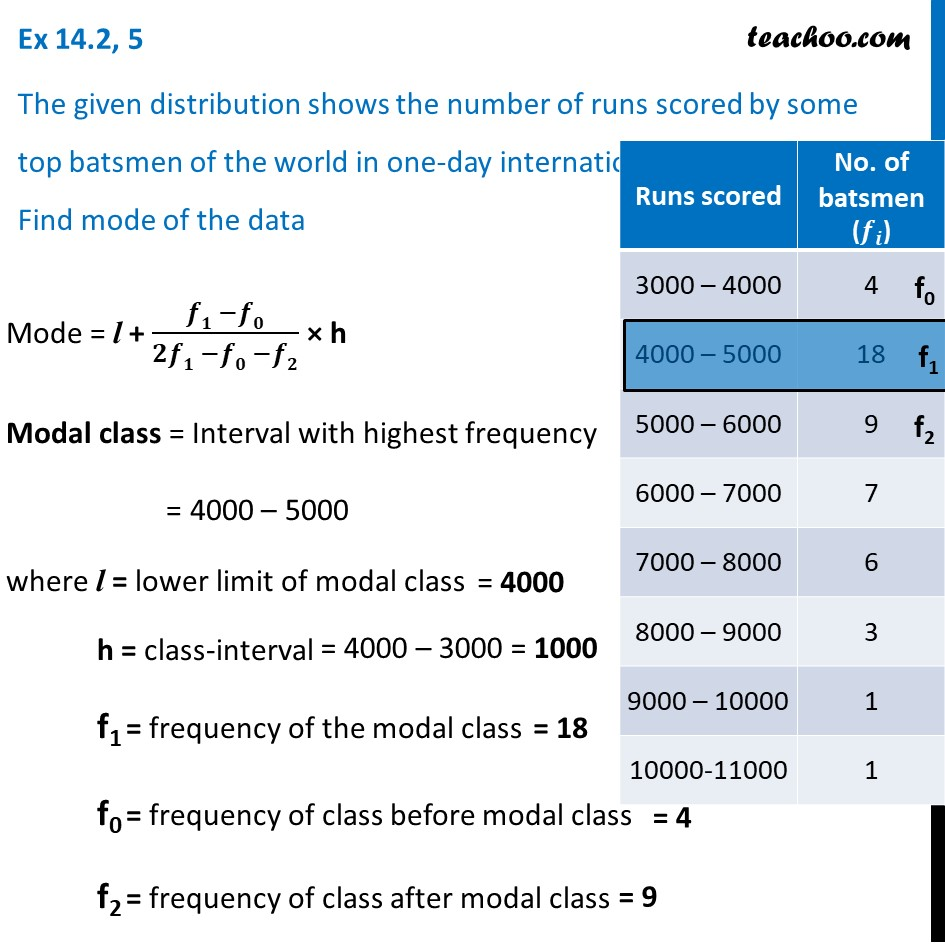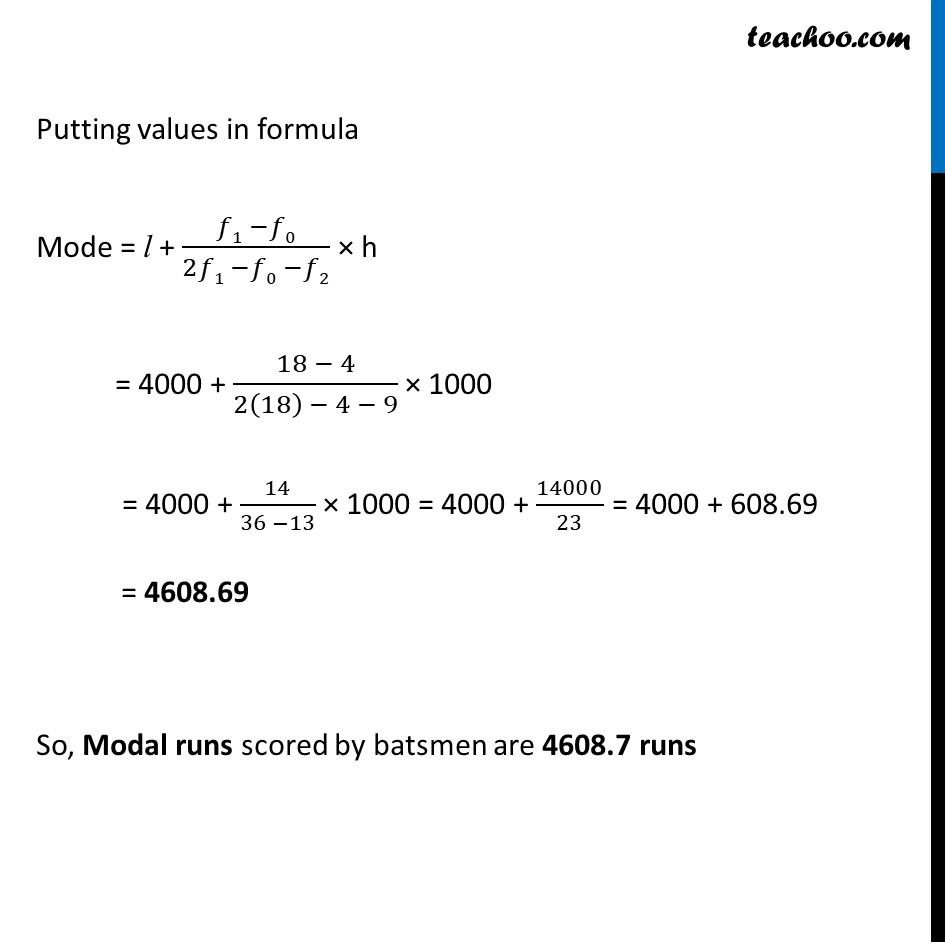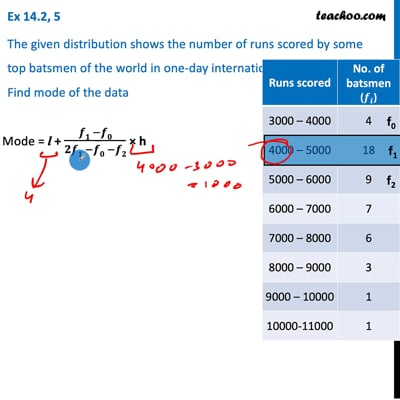Ex 14.2

Chapter 14 Class 10 Statistics
Serial order wiseThis video is only available for Teachoo black users

Introducing your new favourite teacher - Teachoo Black, at only ₹83 per month

### Transcript

Ex 14.2, 5 The given distribution shows the number of runs scored by some top batsmen of the world in one-day international cricket matches. Find mode of the data Mode = l + (𝒇𝟏 −𝒇𝟎)/(𝟐𝒇𝟏 −𝒇𝟎 −𝒇𝟐) × h Modal class = Interval with highest frequency = 4000 – 5000 where l = lower limit of modal class h = class-interval f1 = frequency of the modal class f0 = frequency of class before modal class f2 = frequency of class after modal class Putting values in formula Mode = l + (𝑓1 −𝑓0)/(2𝑓1 −𝑓0 −𝑓2) × h = 4000 + (18 − 4)/(2(18) − 4 − 9) × 1000 = 4000 + 14/(36 −13) × 1000 = 4000 + 14000/23 = 4000 + 608.69 = 4608.69 So, Modal runs scored by batsmen are 4608.7 runs